﻿ 回汽技术在船舶换向中的应用研究
 舰船科学技术2017, Vol. 39Issue (2): 108-113PDF

1. 国家电网黑龙江省电力有限公司发展策划部, 黑龙江 哈尔滨 150001;
2. 中国船舶重工集团公司 第七〇三研究所, 黑龙江 哈尔滨 150001;
3. 哈尔滨工程大学 自动化学院, 黑龙江 哈尔滨 150001

Application of back-steam technology on vessel direction reversing
LIU Gang1, GU Zheng-gang1, WANG Peng2, MENG Hao3
1. Development Planning Department, State Grid Heilongjiang Electric Power Company Limited, Harbin 150001, China;
2. Steam Power Department, Harbin Marine Boiler and Turbine Research Institute Harbin 150001, China;
3. College of Automation, Harbin Engineering University, Harbin 150001, China
Abstract: The astern turbine which exists in marine steam power plant is usually only used in astern condition. In order to prevent the boiler pressure from being overpressure in direction reversing process, it is essential to fully utilize back-steam control. In this paper, we build up mathematical model of steam power plant and propose a boiler-turbine coordinated control system with back-steam control. The simulation results of direction reversing process shows that compared with coordinated control system without back-steam control, the maximum main pressure deviation of boiler-turbine coordinated control system with back-steam technology is 1% less than that of coordinated control system without back-steam technology. The boiler-turbine coordinated control system with back-steam control can provide greater stability of main steam pressure in direction reversing process. It can solve the problem of overpressure in direction reversing process.
Key words: steam power     back-steam control     boiler-turbine coordinated control     ship direction reversing
0 引言

1 船舶蒸汽动力装置的建模

1.1 主蒸汽压力控制系统模型

1.1.1 炉膛

 $\frac{{{Q_r}(s)}}{{M(s)}} = {K_M}{e^{ - {\tau _M}s}}\text{。}$ (1)
1.1.2 蒸发受热面

 $Qdt+D{i_{gs}}dt - D{i_{bq}}dt = {W_b}d{i_{bs}}\text{，}$ (2)

 $\frac{Q}{{{i_{bq}} - {i_{gs}}}} = D+\frac{{{W_b}\frac{{d{i_{bs}}}}{{d{P_b}}}{\rm d}{P_b}}}{{({i_{bq}} - {i_{gs}}){\rm d}t}} \text{，}$ (3)

${C_b} = \frac{{{W_b}\frac{{d{i_{bs}}}}{{d{P_b}}}}}{{{i_{bq}} - {i_{gs}}}}$Cb 为蓄热系数，它表示汽压改变一个单位，蒸发受热面和锅筒所能吞吐的蒸发量。

 ${D_q} = \frac{Q}{{{i_{bq}} - {i_{gs}}}} = D+{C_b}\frac{{{\rm d}{P_b}}}{{{\rm d}t}}\text{，}$ (4)

 ${G_3}(s) = \frac{{{P_b}(s)}}{{{D_q}(s) - D(s)}} = \frac{1}{{{C_b}s}}\text{。}$ (5)
1.1.3 过热器

 $d({P_M} - {P_b}) = 2{K_{gr}}DdD\text{，}$ (6)

$2{K_{gr}}D = {R_{gr}}$，则上式可写成：

 $dD = \frac{{d({P_M} - {P_b})}}{{{R_{gr}}}}\text{，}$ (7)

 ${W_3}(s) = \frac{{D(s)}}{{{P_b}(s) - {P_M}(s)}} = \frac{1}{{{R_{gr}}}}\text{。}$ (8)
1.1.4 母管

 ${W_4}(s) = \frac{{{P_M}(s)}}{{D(s) - {D_T}(s)}} = \frac{1}{{{C_M}s}}\text{。}$ (9)

1.1.5 用汽设备

DT 是母管压力PM，用汽设备背压P0 和调节阀开度μT 的函数。P0 很小且很少变化，假定调节阀为线性的，则用汽设备的传递函数为：

 ${D_T} = \frac{1}{{{R_T}}}{P_M}+{K_T}{\mu _T}\text{，}$ (10)

 ${D_T}(s) = \frac{1}{{{R_T}}}{P_M}(s)+{K_T}{\mu _T}(s)\text{。}$ (11)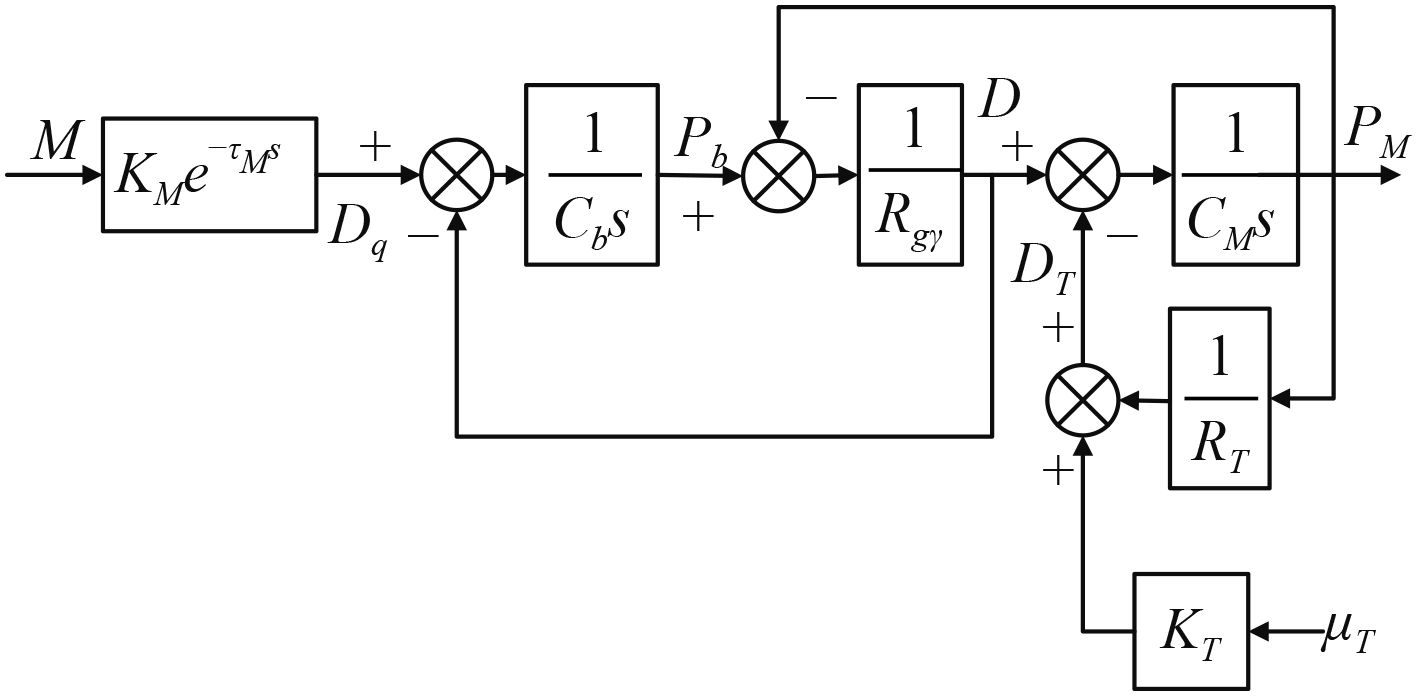图 1 主蒸汽压力被控对象的模型 Fig. 1 Model of main steam pressure
1.2 汽轮机转速控制系统模型

1.2.1 汽轮机本体模型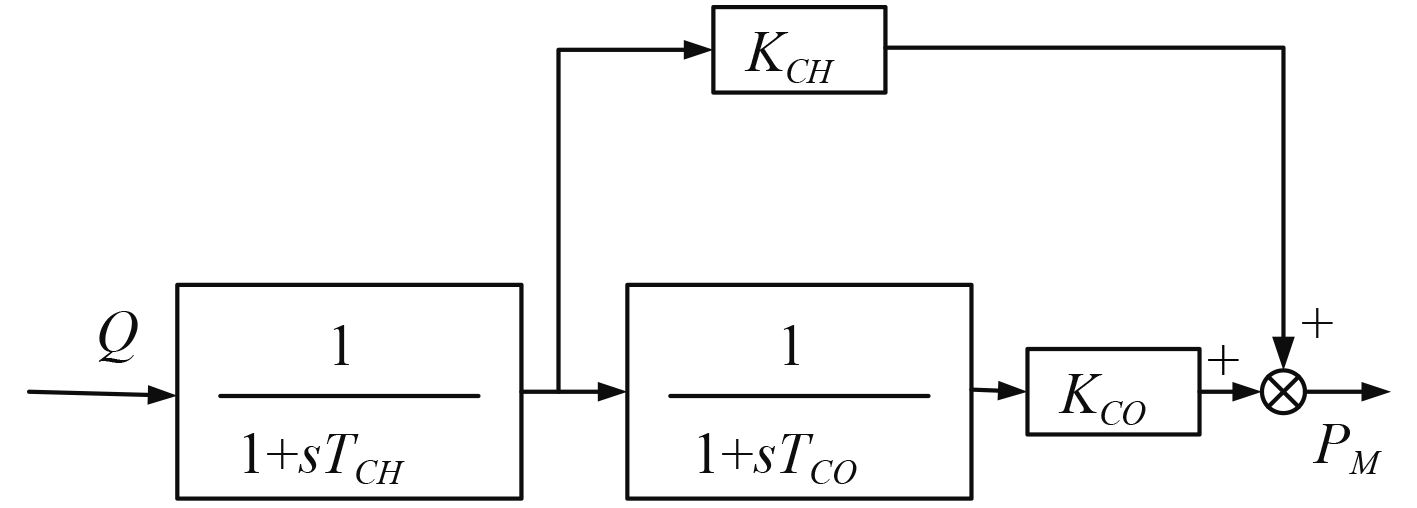图 2 汽轮机模型 Fig. 2 Model of turbine

 $\frac{{{P_M}}}{Q} = \frac{{{K_{CH}}\left( {1+s{T_{CO}}} \right)+{K_{CO}}}}{{\left( {1+s{T_{CH}}} \right)\left( {1+s{T_{CO}}} \right)}}\text{。}$ (12)
1.2.2 汽轮机转速控制机构模型

1） 电液伺服阀模型

 $\frac{P}{I} = \frac{{{K_q}}}{{\frac{{{s^2}}}{{\omega _V^2}}+\frac{{2{\xi _V}}}{{\omega _V}}+1}} = {K_q}\text{。}$ (13)

2） 错油门模型

 $\frac{{{X_V}}}{{{X_i}}} = \frac{{1/A}}{{s\left( {\frac{{{s^2}}}{{\omega {{_h}^2}}}+\frac{{2{\xi _h}s}}{{\omega _h}}+1} \right)}} = K\text{。}$ (14)

3） 错油门输出及油动机模型

 $\frac{S}{L} = \frac{{1/A}}{{s\left( {\frac{{{s^2}}}{{\omega _h^2}}+\frac{{2{\xi _h}}}{{\omega _h}}+1} \right)}}\text{，}$ (15)

 $\frac{S}{L} = \frac{{1/A}}{{s{T_h}+1}}\text{。}$ (16)

4） 蒸汽阀门流量功率模型

 $N = P \times Q = P{C_d}\omega {X_V}\sqrt {\frac{2}{\rho }\Delta P} \text{，}$ (17)

 $N = {K_N}\text{。}$ (18)

1.2.3 汽轮机控制系统负载模型

1） 汽轮机效率及负载扭矩

 $\eta = Ka \times n \text{，}$ (19)

 $T = Kb \times {n^2}\text{。}$ (20)

2） 轴系转动惯量的影响

 $\frac{{{P_{out}}}}{{{P_M}}} = \frac{K}{{1+s{T_z}}}\text{。}$ (21)

3） 蒸汽流量的计算

 $Q = {C_d}\omega {X_v}\Delta P\text{，}$ (22)

4） 汽轮机轴扭矩

 $T = K \times {\omega ^2}\text{，}$ (23)

 $P = \omega \times T\text{。}$ (24)

2 具有回汽控制的机炉协调系统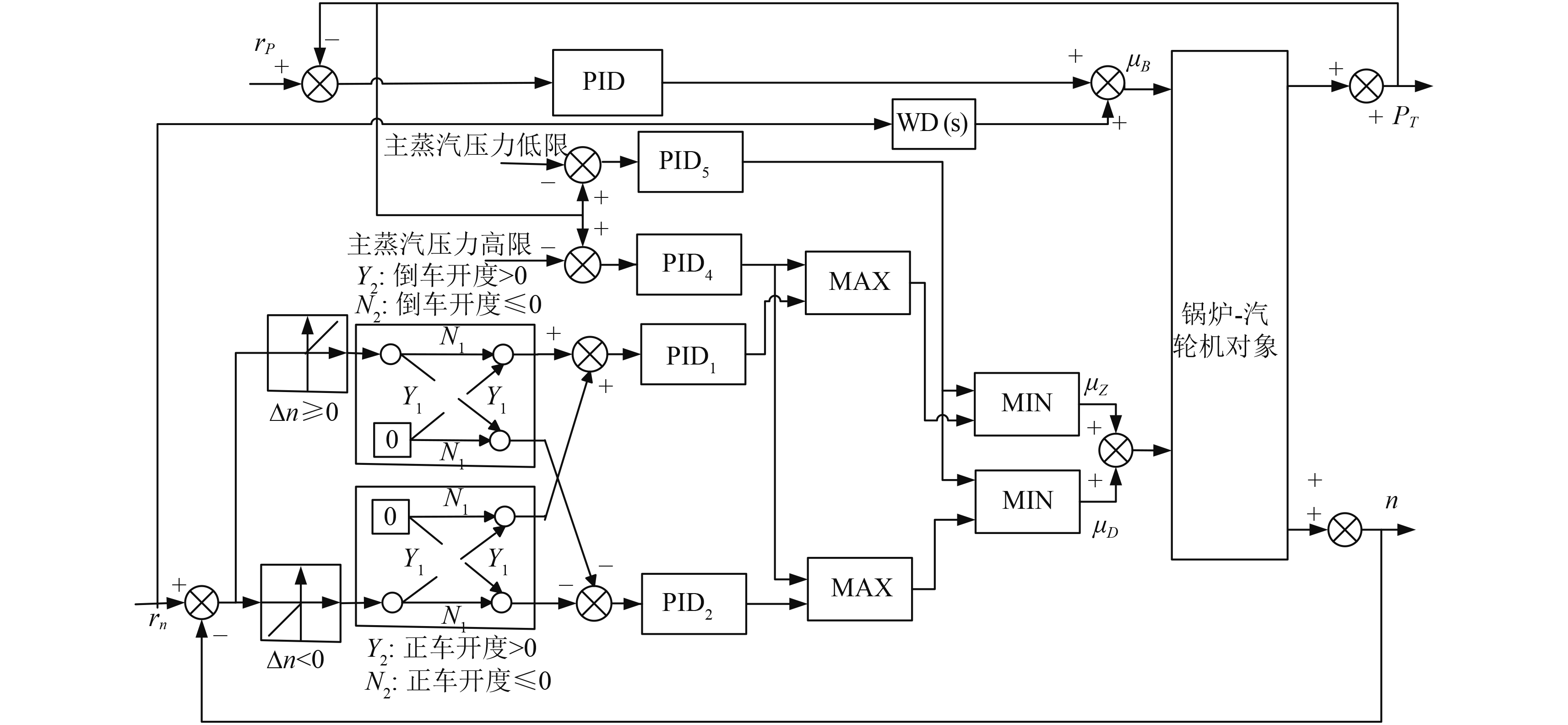图 3 具有回汽控制功能的协调控制系统原理图 Fig. 3 Boiler-turbine coordinated control system with back-steam control technology

rn 信号分为 2 路：一路作为转速控制系统的转速给定信号；另一路引入锅炉主蒸汽压力控制系统，通过前馈环节 WD（s）引入主蒸汽压力控制回路 PID3 控制输出补偿信号，当主机转速给定值变化时，通过前馈环节 WD（s）环节提前改变燃油量，以克服锅炉的惯性，适应主机负荷变化的要求。前馈信号是超前起作用的，当rn 不变化时，前馈环节 WD（s）输出信号保持不变，正好满足要求

3 仿真分析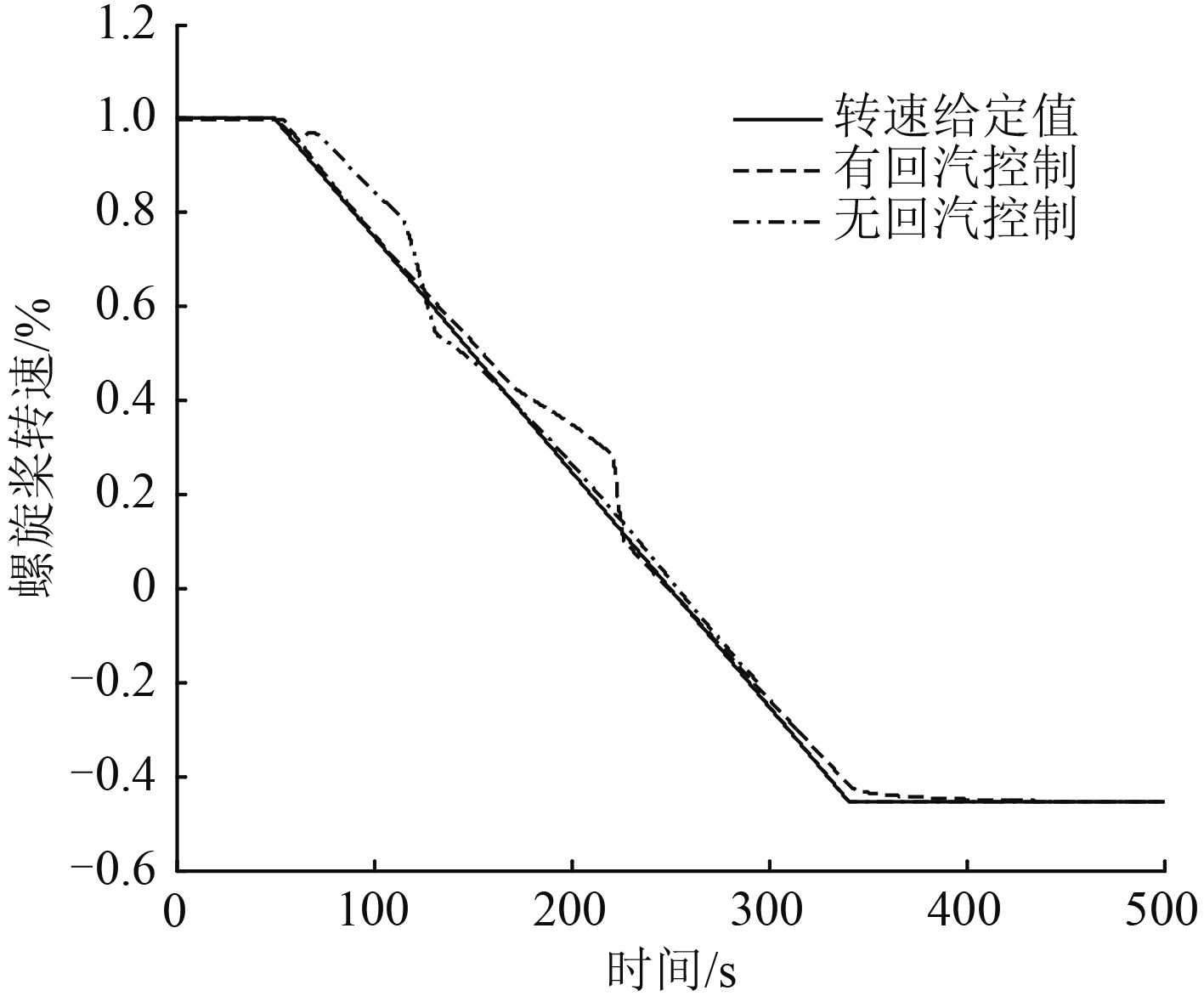图 4 螺旋桨转速 Fig. 4 Rotation speed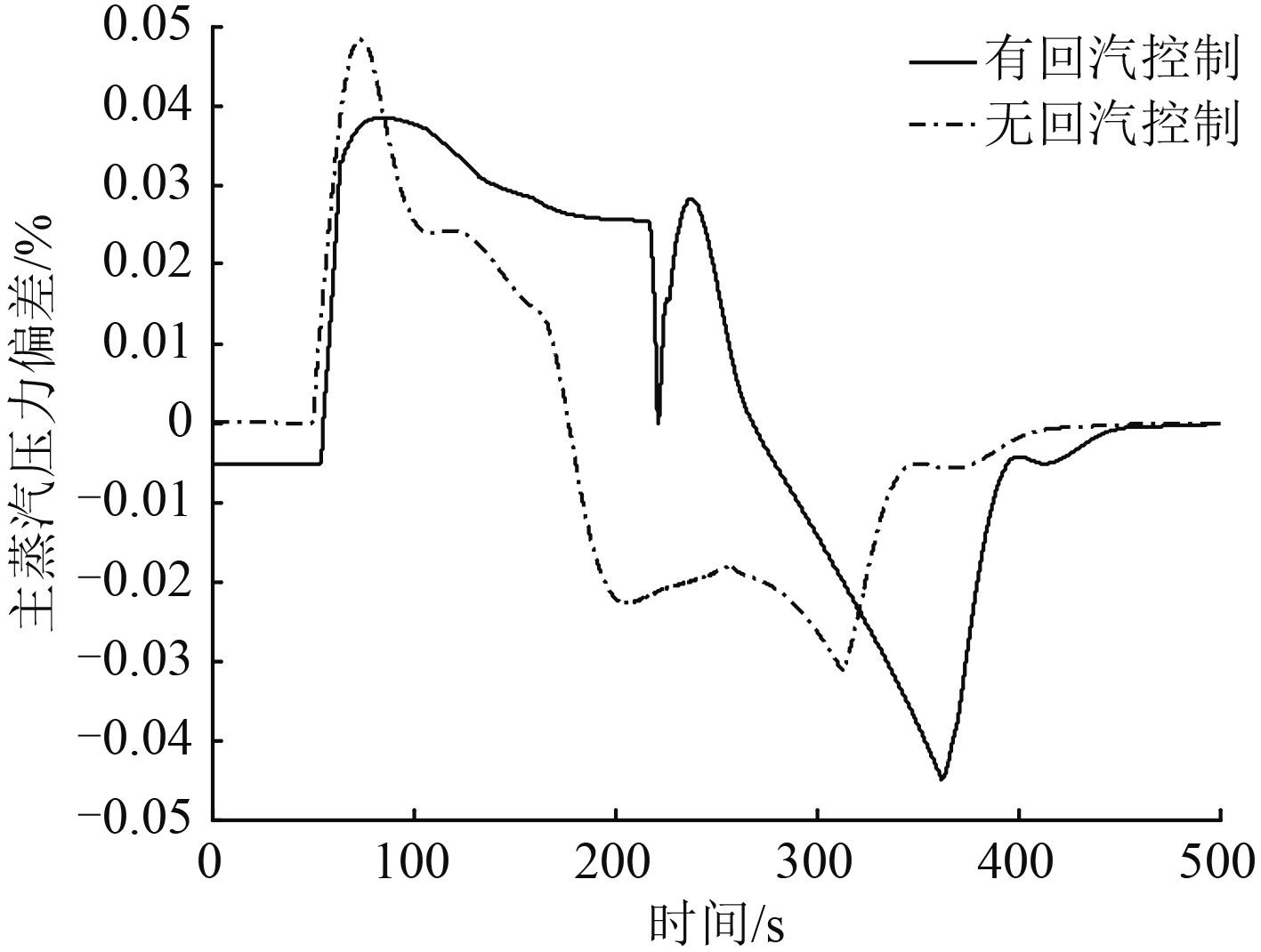图 5 主蒸汽压力偏差 Fig. 5 Main steam pressure deviation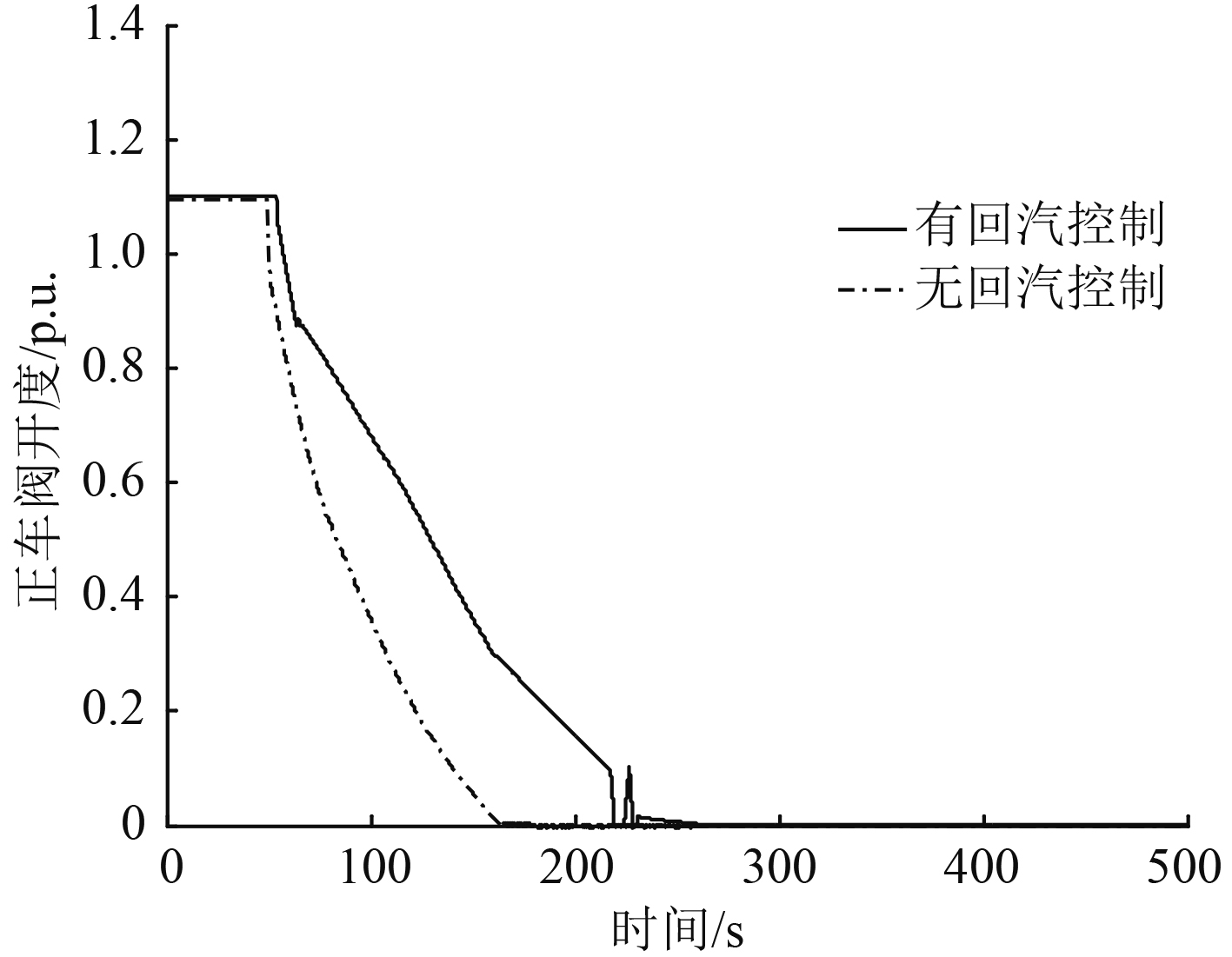图 6 正车阀开度 Fig. 6 Position of ahead valve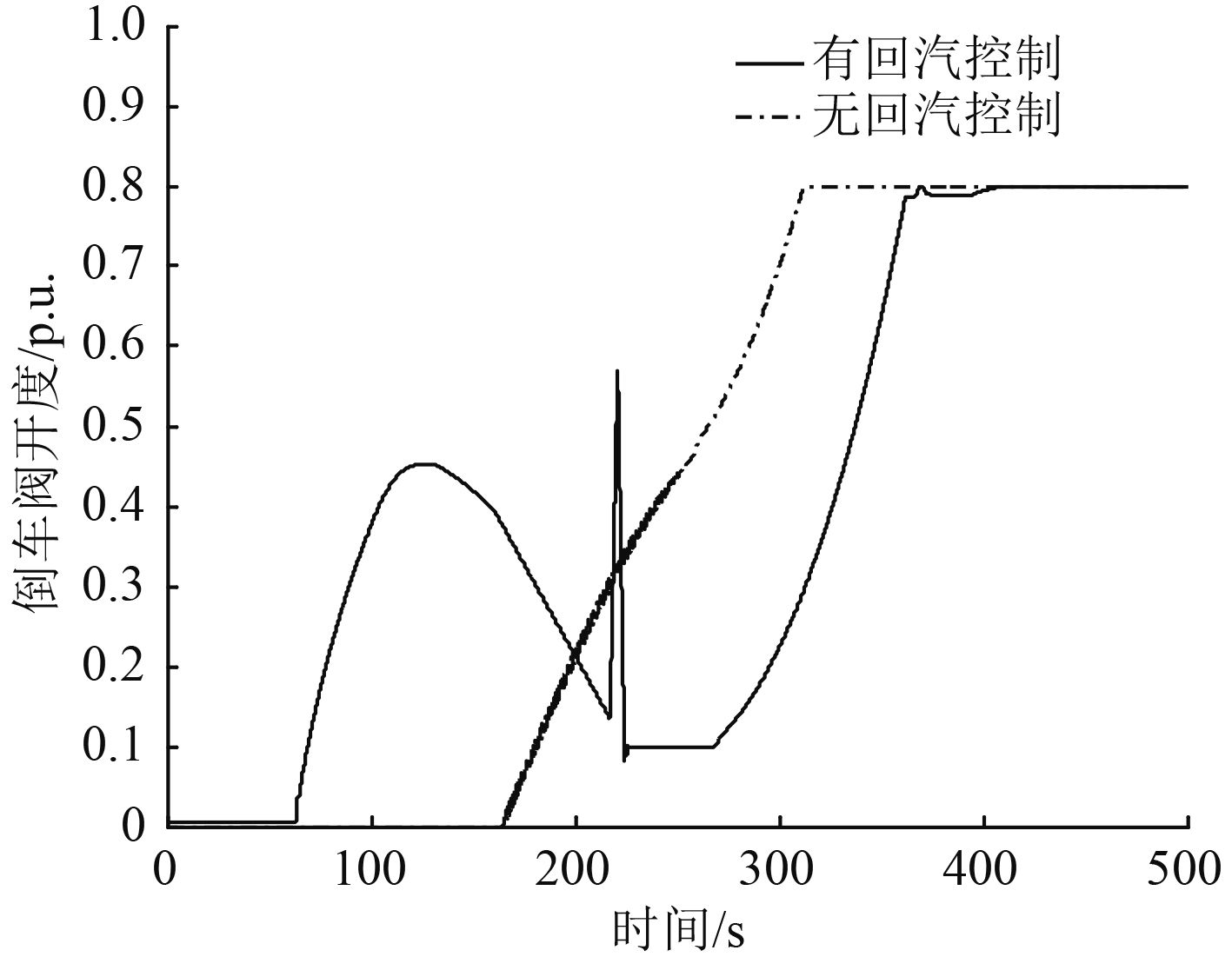图 7 倒车阀开度 Fig. 7 Position of astern valve
4 结语

1） 回汽控制可以有效减小主蒸汽压力的偏差，防止换向过程中可能出现的锅炉超压问题。仿真结果表明，有回汽控制的机炉协调控制的主蒸汽压力偏差最大值要比无回汽控制的机炉协调控制方式小 1%。

2） 回汽控制可以减小正车阀和倒车阀的动作幅度，这对设备的安全有一定好处。

3） 虽然回汽控制可以快速地调节压力，但是回汽的应用是对能量的浪费，过多地使用回汽控制，会影响汽轮机转子的寿命。未来应进一步探究回汽控制的规律，将更多先进的控制方法应用于蒸汽动力装置的回汽控制当中，以求更合理的利用回汽控制技术。

  吕建伟, 易慧, 刘中华. 船舶设计方案评估指标体系研究[J]. 船舶工程, 2005, 27 (4): 53–57. LV Jian-wei, YI Hui, LIU Zhong-hua. Research on index system for evaluating warship Design[J]. Ship Engineering, 2005, 27 (4): 53–57.  朱泳, 金家善, 刘东东. 舰用蒸汽动力装置回汽刹车与回汽保护技术研究[J]. 汽轮机技术, 2012, 54 (6): 404–407.  刘云生, 冯永明, 陈华清, 等. 增压锅炉与涡轮增压机组匹配特性仿真分析[J]. 舰船科学技术, 2012, 4 (1): 50–54.   KECMAN. V. Nonlinearity of dynamic processes in a drum boiler circulation loop[C]//C. Maffezzoni ed. IFAC Modeling and Control of Electric Power Plants. Como, Italy. 1983:21-27.  张文杰, 戚飞虎, 邹文进, 等. 汽轮机建模和仿真研究的一种新方法[J]. 计算机工程, 2001, 27 (9): 175–177. ZHANG Wen-jie, QI Fei-hu, ZHOU Wen-jin, et al. A new method for turbine modularization and simulation research[J]. Computer Engineering, 2001, 27 (9): 175–177.  李东亮, 耿江华, 程刚, 等. 船舶主汽轮机模块化建模方法及实验研究[J]. 舰船科学技术, 2015, 37 (12): 79–82. LI Dong-liang, GENG Jiang-hua, CHENG Gang, et al. Modeling method and simulation experiments of main steam turbine of ship[J]. Ship Science and Technology, 2015, 37 (12): 79–82.  于广. 船舶锅炉蒸汽动力装置多目标控制问题的研究[D]. 哈尔滨:哈尔滨工业大学, 2009:9.  张倩. 船用动力装置机炉协调控制系统优化设计[D]. 哈尔滨:哈尔滨工程大学, 2009:17.  范轶, 沈毅, 徐志强, 于达仁. 船舶蒸汽动力装置调节/保护多目标切换控制问题研究[J]. 汽轮机技术, 2011, 53 (03): 161–163. FAN Yi, SHEN Yi, XU Zhi-qiang, YU Da-ren. Multi-objective regulation and protection switching control for marine steam power system[J]. Turbine Technology, 2011, 53 (03): 161–163.